Question

4. A test has 10 true or false questions. When Rachelle takes the test she answers...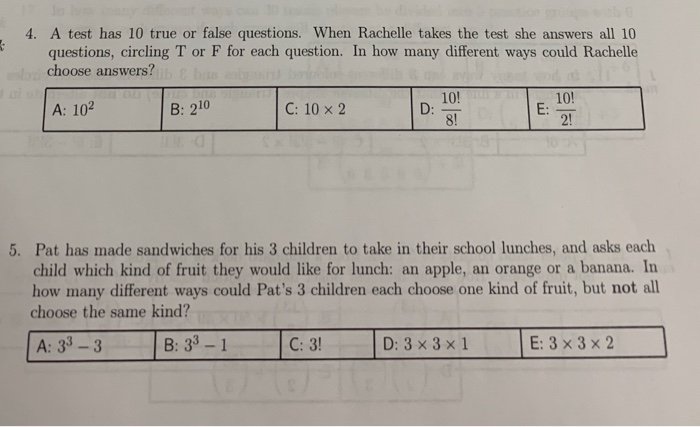4. A test has 10 true or false questions. When Rachelle takes the test she answers all 10 questions, circling T or F for each question. In how many different ways could Rachelle choose answers? 10! A: 102 C: 10 x 2 ID: 8 E : 21 B: 210 5. Pat has made sandwiches for his 3 children to take in their school lunches, and asks each child which kind of fruit they would like for lunch: an apple, an orange or a banana. In how many different ways could Pat's 3 children each choose one kind of fruit, but not all choose the same kind? A: 33 – 3 B: 33 – 1 C: 31 D: 3×3×1 E: 3x3x2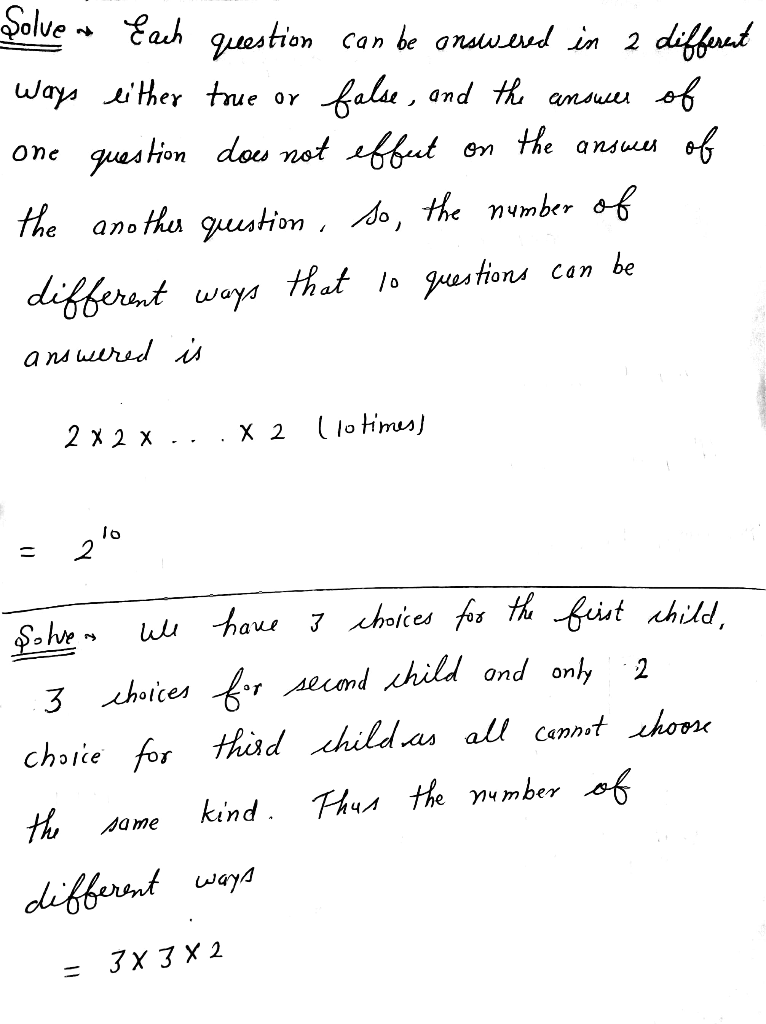Please feel free to ask any query in the comment box and don't forget to rate if you like.

Earn Coins

Coins can be redeemed for fabulous gifts.

Similar Homework Help Questions
• in how many different ways can a true-false test consisting of 9 questions be answers?

in how many different ways can a true-false test consisting of 9 questions be answers?

• please provide the answers clearly 7. Suppose a Truc-False test has 20 questions (a) In how...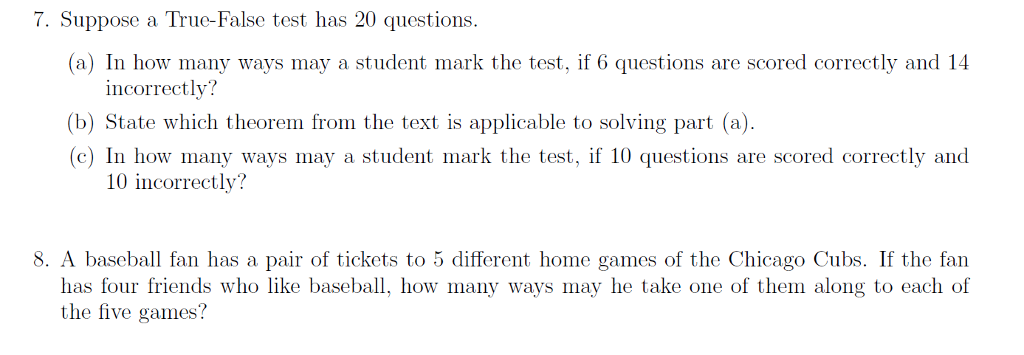please provide the answers clearly 7. Suppose a Truc-False test has 20 questions (a) In how many ways may a student mark the test, if 6 questions are scored correctly and 14 incorrectly? (b) State which theorem from the text is applicable to solving part (a) (c) In how many ways may a student mark the test, if 10 questions are scored correctly and 10 incorrectly? 8. A baseball fan has a pair of tickets to 5 different home games...

• a multiple choice test has 8 questions with 4 possible answers for each question

a multiple choice test has 8 questions with 4 possible answers for each question. if a student were to guess the answer to each question how many different ways would there be to answer the testA. 32B. 4C. 65 536D. 4 096

• how many different ways can twelve questions on a true/false test be answered if a student answers every question

how many different ways can twelve questions on a true/false test be answered if a student answers every question? two different turtors say 24, 12x2, BYU university says 24 is wrong, Help

• A multiple choice test contains 10 questions. There are four possible answers fo...continues

A multiple choice test contains 10 questions. There are four possible answers for each question.(a) How many ways can a student complete the test if every question must be answered?(b) How many ways can a student complete the test if questions can be left unanswered?

• TO SU Part 1: Probability were cay 1. A question on a multiple-choice test has 10...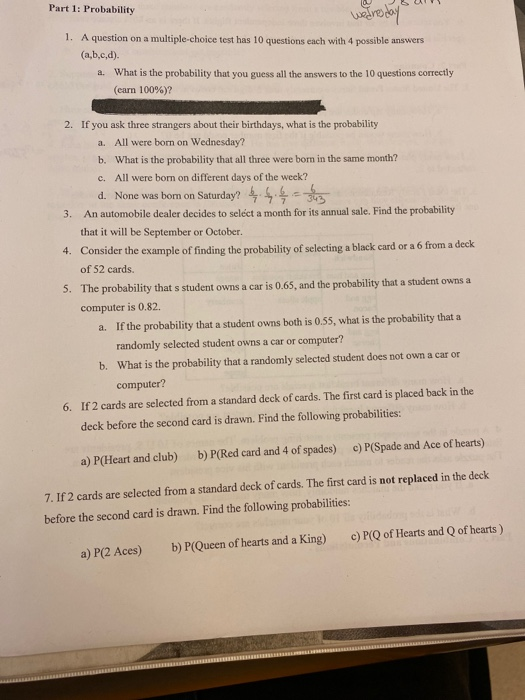TO SU Part 1: Probability were cay 1. A question on a multiple-choice test has 10 questions each with 4 possible answers (a,b,c,d). a. What is the probability that you guess all the answers to the 10 questions correctly (carn 100%)? 2. If you ask three strangers about their birthdays, what is the probability a. All were born on Wednesday? b. What is the probability that all three were born in the same month? c. All were born on different...

• A multiple choice test has 10 questions each of which has 4 possible answers, only one...

A multiple choice test has 10 questions each of which has 4 possible answers, only one of which is correct. If Judy, who forgot to study for the test, guesses on all questions, what is the probability that she will answer exactly 3 questions correctly?

• A test contains 10 multiple-choice questions. Each question has 4 answers and only 1 answer is...

A test contains 10 multiple-choice questions. Each question has 4 answers and only 1 answer is correct. Suppose a student just randomly selects an answer for each question. What is the probability that the student will answer 2 or fewer questions correctly?

• 10. A multiple-choice test has 30 questions and each one has five possible answers, of which one...

10. A multiple-choice test has 30 questions and each one has five possible answers, of which one is correct. If all answers were guesses, find the probability ofgetting exactly four correct answers. (Points : 3).0604.1325.2552.8000

• Mary's Final Exam for Psychology has 10 True/False questions and 10 multiple choice questions with 4 choices for each answer. Assuming Mary randomly guesses on every question: **Write answers usi...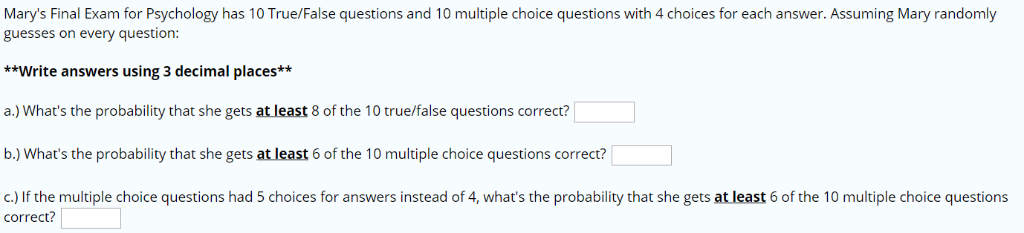Mary's Final Exam for Psychology has 10 True/False questions and 10 multiple choice questions with 4 choices for each answer. Assuming Mary randomly guesses on every question: **Write answers using 3 decimal places* a.) What's the probability that she gets at least 8 of the 10 true/false questions correct? b.) What's the probability that she gets at least 6 of the 10 multiple choice questions correct? c.) If the multiple choice questions had 5 choices for answers instead of 4,...